# Factors influencing rate of reaction

 бет 27/65 Дата 05.11.2016 өлшемі 17,36 Mb.

## - Temperature: Usually conducting a reaction at a higher temperature delivers more energy into the system and increases the reaction rate by causing more collisions between particles, as explained by collision theory. However, the main reason that temperature increases the rate of reaction is that more of the colliding particles will have the necessaryactivation energyresulting in more successful collisions (when bonds are formed between reactants). The influence of temperature is described by theArrhenius equation.

For example, coal burns in a fireplace in the presence of oxygen, but it does not when it is stored at room temperature. The reaction is spontaneous at low and high temperatures but at room temperature its rate is so slow that it is negligible. The increase in temperature, as created by a match, allows the reaction to start and then it heats itself, because it is exothermic. That is valid for many other fuels, such as methanebutane, and hydrogen.

Reaction rates can be independent of temperature (non-Arrhenius) or decrease with increasing temperature (anti-Arrhenius). Reactions without an activation barrier (e.g., someradical reactions), tend to have anti Arrhenius temperature dependence: the rate constant decreases with increasing temperature.

- Solvent: Many reactions take place in solution and the properties of the solvent affect the reaction rate. The ionic strength also has an effect on reaction rate.

- Electromagnetic radiation and intensity of light: Electromagnetic radiation is a form of energy. As such, it may speed up the rate or even make a reaction spontaneous as it provides the particles of the reactants with more energy. This energy is in one way or another stored in the reacting particles (it may break bonds, promote molecules to electronically or vibrationally excited states...) creating intermediate species that react easily. As the intensity of light increases, the particles absorb more energy and hence the rate of reaction increases.

For example, when methane reacts with chlorine in the dark, the reaction rate is very slow. It can be sped up when the mixture is put under diffused light. In bright sunlight, the reaction is explosive.

- A catalyst: The presence of a catalyst increases the reaction rate (in both the forward and reverse reactions) by providing an alternative pathway with a lower activation energy.

For example, platinum catalyzes the combustion of hydrogen with oxygen at room temperature.

- Isotopes: The kinetic isotope effect consists in a different reaction rate for the same molecule if it has different isotopes, usually hydrogen isotopes, because of the relative mass difference between hydrogen and deuterium.

- Surface Area: In reactions on surfaces, which take place for example during heterogeneous catalysis, the rate of reaction increases as the surface area does. That is because more particles of the solid are exposed and can be hit by reactant molecules.

- Stirring: Stirring can have a strong effect on the rate of reaction for heterogeneous reactions.

- Diffusion limit: Some reactions are limited by diffusion.

All the factors that affect a reaction rate, except for concentration and reaction order, are taken into account in the reaction rate coefficient (the coefficient in the rate equation of the reaction).

## Rate equation

Main article: Rate equation

For a chemical reaction a A + b B → p P + q Q, the rate equation or rate law is a mathematical expression used in chemical kinetics to link the rate of a reaction to theconcentration of each reactant. It is of the kind:

{\displaystyle \,r=k(T)[\mathrm {A} ]^{n}[\mathrm {B} ]^{m}}

For gas phase reaction the rate is often alternatively expressed by partial pressures.

In these equations k(T) is the reaction rate coefficient or rate constant, although it is not really a constant, because it includes all the parameters that affect reaction rate, except for concentration, which is explicitly taken into account. Of all the parameters influencing reaction rates, temperature is normally the most important one and is accounted for by the Arrhenius equation.

The exponents n and m are called reaction orders and depend on the reaction mechanism. For elementary (single-step) reactions the order with respect to each reactant is equal to its stoichiometric coefficient. For complex (multistep) reactions, however, this is often not true and the rate equation is determined by the detailed mechanism, as illustrated below for the reaction of H2 and NO.

For elementary reactions or reaction steps, the order and stoichiometric coefficient are both equal to the molecularity or number of molecules participating. For a unimolecular reaction or step the rate is proportional to the concentration of molecules of reactant, so that the rate law is first order. For a bimolecular reaction or step, the number of collisionsis proportional to the product of the two reactant concentrations, or second order. A termolecular step is predicted to be third order, but also very slow as simultaneous collisions of three molecules are rare.

By using the mass balance for the system in which the reaction occurs, an expression for the rate of change in concentration can be derived. For a closed system with constant volume, such an expression can look like

{\displaystyle {\frac {d[\mathrm {P} ]}{dt}}=k(T)[\mathrm {A} ]^{n}[\mathrm {B} ]^{m}}

### Example of a complex reaction: Reaction of hydrogen and nitric oxide

For the reaction

2 H2(g) + 2 NO(g) → N2(g) + 2 H2O(g) the observed rate equation (or rate expression) is: {\displaystyle r=k[\mathrm {H_{2}} ][\mathrm {NO} ]^{2}\,}

As for many reactions, the experimental rate equation does not simply reflect the stoichiometric coefficients in the overall reaction: It is third order overall: first order in H2 and second order in NO, even though the stoichiometric coefficients of both reactants are equal to 2.

In chemical kinetics, the overall reaction rate is often explained using a mechanism consisting of a number of elementary steps. Not all of these steps affect the rate of reaction; normally the slowest elementary step controls the reaction rate. For this example, a possible mechanism is:

1. 2 NO(g) ⇌ N2O2(g) (fast equilibrium)

2. N2O2 + H2 → N2O + H2O (slow)

3. N2O + H2 → N2 + H2O (fast)

Reactions 1 and 3 are very rapid compared to the second, so the slow reaction 2 is the rate determining step. This is a bimolecular elementary reaction whose rate is given by the second order equation:

{\displaystyle r=k_{2}[\mathrm {H_{2}} ][\mathrm {N_{2}O_{2}} ]\,}where k2 is the rate constant for the second step.

However N2O2 is an unstable intermediate whose concentration is determined by the fact that the first step is in equilibrium, so that [N2O2] = K1[NO]2, where K1 is the equilibrium constant of the first step. Substitution of this equation in the previous equation leads to a rate equation expressed in terms of the original reactants

{\displaystyle r=k_{2}K_{1}[\mathrm {H_{2}} ][\mathrm {NO} ]^{2}\,}This agrees with the form of the observed rate equation if it is assumed that k = k2K1. In practice the rate equation is used to suggest possible mechanisms which predict a rate equation in agreement with experiment.

The second molecule of H2 does not appear in the rate equation because it reacts in the third step, which is a rapid step after the rate-determining step, so that it does not affect the overall reaction rate.

## Temperature dependence

Main article: Arrhenius equation

Each reaction rate coefficient k has a temperature dependency, which is usually given by the Arrhenius equation:

{\displaystyle k=Ae^{-{\frac {E_{\mathrm {a} }}{RT}}}}Ea is the activation energy and R is the gas constant. Since at temperature T the molecules have energies given by a Boltzmann distributionone can expect the number of collisions with energy greater than Ea to be proportional to eEaRTA is the pre-exponential factor or frequency factor.

The values for A and Ea are dependent on the reaction. There are also more complex equations possible, which describe temperature dependence of other rate constants that do not follow this pattern.

A chemical reaction takes place only when the reacting particles collide. However, not all collisions are effective in causing the reaction. Products are formed only when the colliding particles possess a certain minimum energy called threshold energy. As a rule of thumb, reaction rates for many reactions double for every 10 degrees Celsius increase in temperature, For a given reaction, the ratio of its rate constant at a higher temperature to its rate constant at a lower temperature is known as its temperature coefficient (Q).Q10 is commonly used as the ratio of rate constants that are 10 °C apart.

## Pressure dependence

The pressure dependence of the rate constant for condensed-phase reactions (i.e., when reactants and products are solids or liquid) is usually sufficiently weak in the range of pressures normally encountered in industry that it is neglected in practice.

The pressure dependence of the rate constant is associated with the activation volume. For the reaction proceeding through an activation-state complex:

A + B ⇌ |A⋯B| → P

the activation volume, ΔV, is:

{\displaystyle \Delta V^{\ddagger }={\bar {V}}_{\ddagger }-{\bar {V}}_{\mathrm {A} }-{\bar {V}}_{\mathrm {B} }}where  denotes the partial molar volumes of the reactants and products and ‡ indicates the activation-state complex.

For the above reaction, one can expect the change of the reaction rate constant (based either on mole-fraction or on molar-concentration) with pressure at constant temperature to be:

{\displaystyle -RT\left({\frac {\partial \ln k_{x}}{\partial P}}\right)_{T}=\Delta V^{\ddagger }}In practice, the matter can be complicated because the partial molar volumes and the activation volume can themselves be a function of pressure.

Reactions can increase or decrease their rates with pressure, depending on the value of ΔV. As an example of the possible magnitude of the pressure effect, some organic reactions were shown to double the reaction rate when the pressure was increased from atmospheric (0.1 MPa) to 50 MPa (which gives ΔV = −0.025 L/mol).

chemical equation

chemical equation is the symbolic representation of a chemical reaction in the form of symbols and formulae, wherein the reactant entities are given on the left-hand side and the product entities on the right-hand side. The coefficients next to the symbols and formulae of entities are the absolute values of the stoichiometric numbers. The first chemical equation was diagrammed by Jean Beguin in 1615.

A chemical equation consists of the chemical formulas of the reactants (the starting substances) and the chemical formula of the products (substances formed in the chemical reaction). The two are separated by an arrow symbol ({\displaystyle \rightarrow }, usually read as "yields") and each individual substance's chemical formula is separated from others by a plus sign.

As an example, the equation for the reaction of hydrochloric acid with sodium can be denoted:

2 HCl + 2 Na → 2 NaCl + H

2

This equation would be read as "two HCl plus two Na yields two NaCl and H two." But, for equations involving complex chemicals, rather than reading the letter and its subscript, the chemical formulas are read using IUPAC nomenclature. Using IUPAC nomenclature, this equation would be read as "hydrochloric acid plus sodium yields sodium chloride andhydrogen gas."

This equation indicates that sodium and HCl react to form NaCl and H2. It also indicates that two sodium molecules are required for every two hydrochloric acid molecules and the reaction will form two sodium chloride molecules and one diatomic molecule of hydrogen gas molecule for every two hydrochloric acid and two sodium molecules that react. Thestoichiometric coefficients (the numbers in front of the chemical formulas) result from the law of conservation of mass and the law of conservation of charge (see "Balancing Chemical Equation" section below for more information).

## Common symbols

Symbols are used to differentiate between different types of reactions. To denote the type of reaction:

• "{\displaystyle =}=" symbol is used to denote a stoichiometric relation.

• "{\displaystyle \rightarrow }→" symbol is used to denote a net forward reaction.

• "{\displaystyle \rightleftarrows }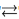" symbol is used to denote a reaction in both directions.

• "{\displaystyle \rightleftharpoons }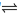" symbol is used to denote an equilibrium.

The physical state of chemicals is also very commonly stated in parentheses after the chemical symbol, especially for ionic reactions. When stating physical state, (s) denotes a solid, (l) denotes a liquid, (g) denotes a gas and (aq) denotes an aqueous solution.

If the reaction requires energy, it is indicated above the arrow. A capital Greek letter delta ({\displaystyle \Delta }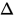) is put on the reaction arrow to show that energy in the form of heat is added to the reaction. {\displaystyle h\nu }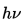is used if the energy is added in the form of light. Other symbols are used for other specific types of energy or radiation.

## Balancing chemical equations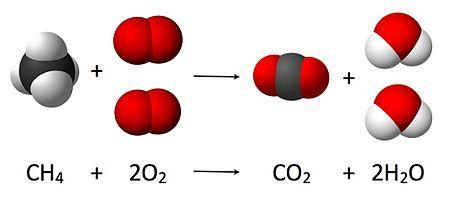As seen from the equation CH

4 + 2 O

2 → CO

2 + 2 H

2O, a coefficient of 2 must be placed before the oxygen gas on the reactants side and before the water on the products side in order for, as per the law of conservation of mass, the quantity of each element does not change during the reaction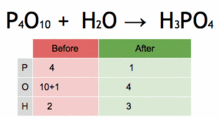P4O10 + 6 H2O → 4 H3PO4

This chemical equation is being balanced by first multiplying H3PO4 by four to match the number of P atoms, and then multiplying H2O by six to match the numbers of H and O atoms.

The law of conservation of mass dictates that the quantity of each element does not change in a chemical reaction. Thus, each side of the chemical equation must represent the same quantity of any particular element. Likewise, the charge is conserved in a chemical reaction. Therefore, the same charge must be present on both sides of the balanced equation.

One balances a chemical equation by changing the scalar number for each chemical formula. Simple chemical equations can be balanced by inspection, that is, by trial and error. Another technique involves solving a system of linear equations.

Balanced equations are written with smallest whole-number coefficients. If there is no coefficient before a chemical formula, the coefficient 1 is understood.

The method of inspection can be outlined as putting a coefficient of 1 in front of the most complex chemical formula and putting the other coefficients before everything else such that both sides of the arrows have the same number of each atom. If any fractional coefficient exists, multiply every coefficient with the smallest number required to make them whole, typically the denominator of the fractional coefficient for a reaction with a single fractional coefficient.

As an example, seen in the above image, the burning of methane would be balanced by putting a coefficient of 1 before the CH4:

1 CH4 + O2 → CO2 + H2O

Since there is one carbon on each side of the arrow, the first atom (carbon) is balanced.

Looking at the next atom (hydrogen), the right-hand side has two atoms, while the left-hand side has four. To balance the hydrogens, 2 goes in front of the H2O, which yields:

1 CH4 + O2 → CO2 + 2 H2O

Inspection of the last atom to be balanced (oxygen) shows that the right-hand side has four atoms, while the left-hand side has two. It can be balanced by putting a 2 before O2, giving the balanced equation:

CH4 + 2 O2 → CO2 + 2 H2O

This equation does not have any coefficients in front of CH4 and CO2, since a coefficient of 1 is dropped.

Достарыңызбен бөлісу:

©engime.org 2020
әкімшілігінің қараңыз

Басты бет